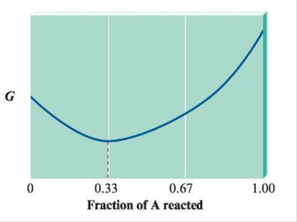# Consider the following diagram of free energy ( G ) versus fraction of A reacted in terms of moles for the reaction 2A( g ) →B( g ). Before any A has reacted, P A = 3.0 atm and P B = 0. Determine the sign of ∆G° and the value of K p . for this reaction.### Chemistry: An Atoms First Approach

2nd Edition
Steven S. Zumdahl + 1 other
Publisher: Cengage Learning
ISBN: 9781305079243

#### Solutions

Chapter
Section### Chemistry: An Atoms First Approach

2nd Edition
Steven S. Zumdahl + 1 other
Publisher: Cengage Learning
ISBN: 9781305079243
Chapter 16, Problem 94AE
Textbook Problem
1 views

## Consider the following diagram of free energy (G) versus fraction of A reacted in terms of moles for the reaction 2A(g) →B(g).Before any A has reacted, PA = 3.0 atm and PB = 0. Determine the sign of ∆G° and the value of Kp. for this reaction.

Interpretation Introduction

Interpretation: The diagram of free energy versus fraction of A and partial pressure of A and B before any A has reacted is given. The sign of ΔG° and the value of Kp is to be calculated.

Concept introduction: Equilibrium constant K , is defined as the ratio of the concentration of products to that of the reactants at equilibrium. If a given reaction is at equilibrium, the free energy change is,

ΔG=0Q=K

If the partial pressure of reactant and product is given, then the equilibrium pressure is expressed as Kp . The expression for free energy change is,

ΔG°=RTln(Kp)

### Explanation of Solution

Given

Before any A has reacted, the partial pressure of A , PA , is 3.0atm .

Before any A has reacted, the partial pressure of B , PB , is 0atm .

The stated reaction is,

2A(g)B(g)

The given graph is,

Figure 1

The diagram shows that equilibrium is closer to the reactant. The pressure of reactant A is 0.33 times the original pressure. Hence, the total pressure of A is,

0.33(3.0atm)=0.99atm

If the partial pressure of reactant and product is given, then the equilibrium pressure is expressed as Kp and its expression is written as,

Kp=(Partialpressure of product)a(Partialpressure of reactant)b

Where,

• a is the number of moles of product

### Still sussing out bartleby?

Check out a sample textbook solution.

See a sample solution

#### The Solution to Your Study Problems

Bartleby provides explanations to thousands of textbook problems written by our experts, many with advanced degrees!

Get Started

Find more solutions based on key concepts
The smallest unit of any substance is the _____. a. atom b. molecule c. cell

Biology: The Unity and Diversity of Life (MindTap Course List)

Energy-yielding nutrients include all of the following except. a. vitamins b. carbohydrates c. fat d. protein

Nutrition: Concepts and Controversies - Standalone book (MindTap Course List)

Write the correct IUPAC name for each of the following: a. b. c. d. e.

Chemistry for Today: General, Organic, and Biochemistry

Why are some genes expressed and some not?

BIOLOGY:CONCEPTS+APPL.(LOOSELEAF)

How is waters density affected by freezing? Why does ice float?

Oceanography: An Invitation To Marine Science, Loose-leaf Versin

Discuss the similarities between the energy stored in the electric field of a charged capacitor and the energy ...

Physics for Scientists and Engineers, Technology Update (No access codes included)